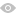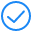## RESOURCES

Current Position: Home>>RESOURCES>>Protocols>>Biochemical detection >>Reaction principle of biochemical test kit

# Reaction principle of biochemical test kit

1 Reaction curve.

Firstly, let's take a look at the time and absorbance of a chemical reaction curve figure. It can be divided into four regions: the delay region, the constant velocity region, the transition region and the equilibrium region, as shown in the following figure:2 Reaction principle.

Delay region is senseless to test indicator and without any law.

The indicator detection method in the constant velocity zone is the rate method. The rate method, also known as the continuous monitoring method, is to continuously select four or more photometric points in the linear period of the time-absorbance curve as the reading point and calculate the value via the absorbance change value per unit time. When measuring the enzyme activity or determining the metabolite by enzymatic method. The linear phase is the difference in absorbance between the various photometric points during this period, the substrate of the enzymatic reaction is a zero-order reaction. The photometric point is generally set after the start-up factor is added and incubated for a period, last for 1-3 min, the continuous monitoring method is generally applied to the enzyme project, and the factor method can be used to calculate the concentration.Schematic diagram of biochemical detection principle

The transition region corresponding to the fixed-time method. A special case in the endpoint method, refers to choose two reading points on the time-absorbance curve. These two points are neither the reaction initial absorbance nor the equilibrium spot absorbance. The difference of the absorbance of these two points is used to calculate the result. The goal is to solve certain non-specific problems about chemical reactions and improve accuracy.

According to the number of light-measuring points, the balance region corresponding to the end point method can be divided into one-point termination method and two-point termination method.

The one-point termination method is to select a light-measuring point to calculate the concentration of the substance to be tested when the reaction reaches the equilibrium point, that is, the absorbance does not change on the time-absorbance curve.

The two-point termination method is often used in the two-reagent assay. Most of the first reagents usually contain only buffers and other components. They generally do not react specifically with the sample; therefore, before the second reagent is added, select a light-measuring as the first reading point, the absorbance at this time is equivalent to the sample blank. After the second reagent is added, the substance to be tested reacts and reaches an equilibrium point after a certain period. At this time, select the second reading point , and calculate the concentration of the substance by the difference between the absorbance of the two reading points .The two-point method can effectively eliminate the light absorption interference caused by hemolysis, jaundice, and turbidity of the sample. Such as enzymatic determination of glucose, total cholesterol, triglycerides, uric acid, creatinine, and chemical methods for determination of total protein, total bilirubin, direct bilirubin, calcium, phosphorus, magnesium, iron, etc.; and reach the end of the reaction  within 2-5 min after adding the second reagent.

• Reviews
Rating:

Create new Reviews (You should Login first before Submitting)

VerifyCode### Leave Message

*
*
*
*
*
*
*
Click to load CaptchaMessage submitted successfully !